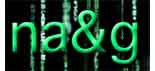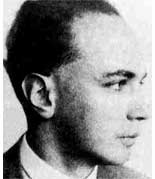# Tag: modularTomorrow
in our masterclass. The idea of this course (and its companion
Projects in non-commutative algebra run by Fred Van Oystaeyen) is
that students should make a small (original if possible) work, that may
At this moment the students
have seen the following : definition and examples of quasi-free algebras
(aka formally smooth algebras, non-commutative curves), their
representation varieties, their connected component semigroup and the
Euler-form on it. Last week, Markus Reineke used all this in his mini-course
Rational points of varieties associated to quasi-free
algebras
. In it, Markus gave a method to compute (at least in
principle) the number of points of the non-commutative Hilbert
scheme
and the varieties of simple representations over a
finite field. Here, in principle means that Markus demands a lot of
knowledge in advance : the number of points of all connected components
of all representation schemes of the algebra as well as of its scalar
extensions over finite field extensions, together with the action of the
Galois group on them … Sadly, I do not know too many examples were all
this information is known (apart from path algebras of quivers).
Therefore, it seems like a good idea to run through Markus’
calculations in some specific examples were I think one can get all this
: free products of semi-simple algebras. The motivating examples
being the groupalgebra of the (projective) modular group
PSL(2,Z) = Z(2) * Z(3) and the free matrix-products $M(n,F_q) * M(m,F_q)$. I will explain how one begins to compute things in these
examples and will also explain how to get the One
quiver to rule them all
in these cases. It would be interesting to
compare the calculations we will find with those corresponding to the
path algebra of this one quiver.
As Markus set the good
example of writing out his notes and posting them, I will try to do the
same for my previous two sessions on quasi-free algebras over the next
couple of weeks.Non-commutative geometry seems pretty trivial compared
to commutative geometry : there are just two types of manifolds,
points and curves. However, nobody knows how to start classifying
these non-commutative curves. I do have a conjecture that any
non-commutative curve can (up to non-commutative birationality) be
constructed from hereditary orders over commutative curves
by universal methods but I’ll try to explain that another
time.

On the other hand, non-commutative points
have been classified (at least in principle) for at least 50
years over an arbitrary basefield $l$. non-commutative
$l$-points $P$ is an $l$-algebra such that its double
$d(P) = P \\otimes P^o$ ( where $P^o$ is the opposite algebra,
that is with the reverse multiplication) has an element$c=\\sum_i a_i \\otimes b_i with \\sum_i a_ib_i = 1 (in$P$)$ and such that for
all p in $P$ we have that $(1 \\otimes a).c = (a \\otimes 1).c$ For
people of my generation, c is called a separability idempotent
and $P$ itself is called a separable $l$-algebra.
Examples of $l$-points include direct sums of full matrixrings
(of varying sizes) over $l$ or group-algebras $lG$ for $G$ a
finite group of n elements where n is invertible in $l$. Hence, in
particular, the group-algebra $lG$ of a p-group $G$ over a field $l$
of characteristic p is a non-commutative singular point and
modular representation theory (a theory build almost single
handed by
Richard Brauer) can be viewed as
the methods needed to resolve this singularity. Brauer’s name is
still mentioned a lot in modular representation theory, but another
of his inventions, the Brauer group, seems to be hardly known
among youngsters.

Still, it is a crucial tool
in classifying all non-commutative $l$-points. The algebraic
structure of an $l$-point $P$ is as follows : $$P = S_1 + S_2 + … + S_k$$ where each S_i is a simple algebra (that is, it
contains no proper twosided ideals), finite dimensional over
its center $l_i$ which is in its turn a finite dimensional
separable field extension of $l$. So we need to know all
simple algebras $S$, finite dimensional over their center $L$ which
is any finite dimensional separable field extension of $l$. The
algebraic structure of such an $S$ is of the form$$S = M(a,D)$$ that
is, full axa matrices with entries in $D$ where $D$ is a
skew-field (or some say, a division algebra) with
center $L$. The $L$-dimension of such a $D$ is always a square,
say b^2, so that the $L$-dimension of $S$ itself is also a square
a^2b^2. There are usually plenty such division algebras, the simplest
examples being quaternion algebras. Let p and q be two
non-zero elements of $L$ such that the conic $C : X^2-pY^2-bZ^2 = 0$ has no $L$-points in the projective $L$-plane, then the
algebra$D=(p,q)_2 = L.1 + L.i + L.j + L.ij where i^2=p, j^2=q and ji=-ij$ is a four-dimensional skew-field over $L$. Brauer’s idea to
classify all simple $L$-algebras was to associate a group to them,
the Brauer group, $Br(L)$. Its elements are equivalence
classes
of simple algebras where two simple algebras $S$ and
$S’$ are equivalent if and only if$M(m,S) = M(n,S’)$ for some sizes
m and n. Multiplication on these classes in induced by
the tensor-product (over $L$) as $S_1 \\otimes S_2$ is again a simple
$L$-algebra if $S_1$ and $S_2$ are. The Brauer group $Br(L)$ is an
Abelian torsion group and if we know its structure we know all
$L$-simple algebras so if we know $Br(L)$ for all finite dimensional
separable extensions $L$ of $l$ we have a full classification of
all non-commutative $l$-points.

Here are some examples
of Brauer groups : if $L$ is algebraically closed (or separable
closed), then $Br(L)=0$ so in particular, if $l$ is algebraically
closed, then the only non-commutative points are sums of matrix rings.
If $R$ is the field of real numbers, then $Br(R) = Z/2Z$ generated by
the Hamilton quaternion algebra (-1,-1)_2. If $L$ is a complete
valued number field, then $Br(L)=Q/Z$ which allows to describe also
the Brauer group of a number field in terms of its places. Brauer groups
of function fields of (commutative) varieties over an algebraically
closed basefield is usually huge but there is one noteworthy
exception $Tsen’s theorem$ which states that $Br(L)=0$ if $L$ is the
function field of a curve C over an algebraically closed field. In 1982
Merkurjev and Suslin proved a marvelous result about generators of
$Br(L)$ whenever $L$ is large enough to contain all primitive roots
of unity. They showed, in present day lingo, that $Br(L)$
is generated by non-commutative points of the quantum-planes
over $L$ at roots of unity. That is, it is generated by cyclic
algebras
of the form$(p,q)_n = L \\< X,Y>/(X^n=p,Y^n=q,YX=zXY)$where z is an n-th primitive root of
unity. Next time we will recall some basic results on the relation
between the Brauer group and Galois cohomology.